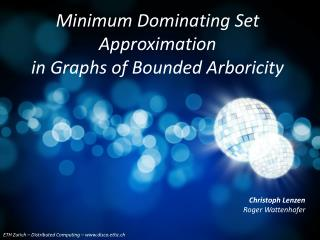DownloadDownload PresentationMinimum Dominating Set Approximation in Graphs of Bounded Arboricity

Minimum Dominating Set Approximation in Graphs of Bounded Arboricity

Download PresentationMinimum Dominating Set Approximation in Graphs of Bounded Arboricity

- - - - - - - - - - - - - - - - - - - - - - - - - - - E N D - - - - - - - - - - - - - - - - - - - - - - - - - - -
Presentation Transcript

1. Minimum Dominating Set Approximation in Graphs of Bounded Arboricity

2. Minimum Dominating Sets (MDS) • important in theory and practice minimum dominating set dominating set in a social network • graph G=(V,E) • N(A) denotes inclusive neighborhood of AµV • DµV is dominating set (DS) iff V=N(D) • minimum dominating set is DS of minimum size

3. MDS on General Graphs • finding an MDS is NP-hard • ) we're looking for approximations • O(log Δ) approx. in O(log n) rounds • ...but for reasonable message size O(log2Δ) rounds • o(log Δ) approx. is NP-hard • polylog. approx. needs (log Δ)and (log1/2 n) rounds • ) maybe "simpler" graphs are easier? Kuhn & al., SODA '06 Garey & Johnson, '79 Raz & Safra, STOC '97 Feige, JACM '98 Kuhn & al., PODC '04

4. MDS on Restricted Families of Graphs excluded minor Schneider & Wattenhofer, PODC '08 bounded independence hard restrictive L. et al DISC '08 planar O(1) approx. O(1) rounds (1+²) approx. polylog n rounds general bounded degree Θ(log n) approx. O(log2Δ) rounds (log Δ) rounds O(1) approx. O(1) rounds unit disc O(1) approx. O(log n) rounds O(1) approx. Θ(log*n) rounds L. et al SPAA '08 e.g. Luby SIAM J. Comp. '86 Czygrinow & Hańćkowiak, ESA '06

5. What's a Good Compromise? • ...or: what have many "easy" graphs in common? • ) They are sparse! • This is not good enough: O(n) edges = + same lower bounds as in general case arbitrary graph: n1/2 nodes difficult to handle star graph: n-n1/2 nodes center covers all

6. Arboricity • A "good" property is preserved under taking subgraphs. • ) Demand sparsity in every subgraph! • This property is called bounded arboricity. 3-forest decomp. of the Peterson graph... ...whose arboricity is however only 2. • graph G=(V,E) • partition E=E1 [E2 [...[Ef into f forests • minimum number of forests is arboricityA of G

7. Where are Graphs of Bounded Arboricity? bounded independence hard restrictive no o(A) approx. in o(log* n) rounds • arboricity 2 permits K√n minor • no strong lower bounds • o(log A) approx. is NP-hard • no (5-²) approximation in o(log* n) time bounded arboricity bounded arboricity excluded minor planar general bounded degree unit disc Czygrinow & al., DISC '08

8. Be Greedy! 2 4 5 8+2 Θ(log n) 5 1 2 4 7+2 3 1 4 1 7+2 3 • sequentially add nodes covering most others • ) yields O(log Δ) approx. • ...but in parallel? • ) Just take all high-degree nodes! • repeat until finished

9. Why does Greedy-By-Degree work? V • D = nodes of (current) max. deg. Δ • C = nodes (freshly) covered by D • M = optimum solution • |D|Δ/2 · |E(C[D)| < A(|C[D|) · A(|C|+|D|) • ) (Δ/2-A)|D| < A|C| · A(Δ+1)|M| • if Δ¸ 4A and A 2 O(1) • ) |D| 2 O(|M|) D C M

10. Greedy-By-Degree: Details Q: What about Δ < 4A ? A: Each c2C elects one deg. Δ neighbor into D! Q: How avoid time complexity (Δ)? A: Take all nodes of degree Δ/2 at once! Q: How deal with unknown Δ? A: It's enough to check up to distance 2! ) uniform O(log Δ) approx. in O(log Δ) rounds

11. Neat, but... • ...we would like to have an O(1) approx. for A 2 O(1) • What about using a (rooted) forest decomposition? • decomposition into f 2 O(A) forests takes Θ(log n) time • note: we cannot handle each forest individually Barenboim & Elkin, PODC '08

12. How to use a Forest-Decomposition {6} 1 {1,3,7} {9} {1,10} 6 2 5 {9,10} 7 10 {3,6,10} 9 8 {3,5,9} 3 4 • For an MDS M, ·(A+1)|M| nodes are not covered by parents. • ) These have ·A(A+1)|M| parents. • ) Let's try to cover all nodes (that have one) by parents! • ) set cover instance with each element in · A sets )

13. Acting Greedily again • sequentially, an A approx. is trivial: • pick any uncovered node • choose all of its parents • repeat until finished • for every node, one of its parents is in an optimum solution {6} 1 {1,3,7} {9} {1,10} 6 2 5 {9,10} 7 10 {3,6,10} 9 8 {3,5,9} 3 4

14. And now more quickly... ) • any sequence of nodes that share no parents is feasible • the order is irrelevant for the outcome • define H:=(V,E') by {v,w} 2 E' , v and w share a parent • ) we need a maximal independent in H

15. Algorithm: Parent Dominating Set ) • compute O(A) forest decomp. (O(log n) rounds) • simulate MIS algorithm on H (O(log n) rounds w.h.p. • output parents of MIS nodes and nodes w/o parents • ) O(A2) approx. in O(log n) rounds w.h.p.

16. Greedy-By-Degree: Pros'n'Cons general graphs: O(log2Δ) + very simple + running timeO(log Δ) + message size O(log log Δ) + uniform & deterministic - O(A log Δ) approx. general graphs: O(log Δ)

17. Parent Dominating Set: Pros'n'Cons ) general graphs: O(log Δ) • + simple • + O(A2) approx. (deterministic) • +/- running time O(log n) (randomized) • open question: • Are there faster O(1) approx. for A2O(1)?